# Chapter 18 Electric Charges and Forces The electric

• Slides: 52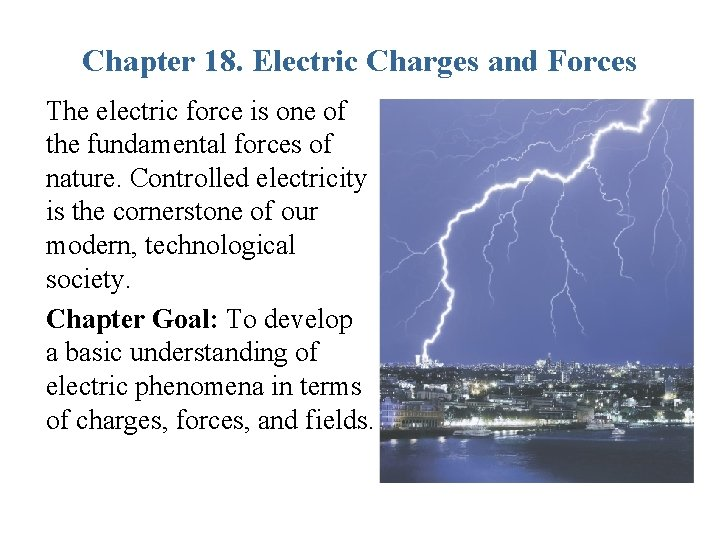Chapter 18. Electric Charges and Forces The electric force is one of the fundamental forces of nature. Controlled electricity is the cornerstone of our modern, technological society. Chapter Goal: To develop a basic understanding of electric phenomena in terms of charges, forces, and fields.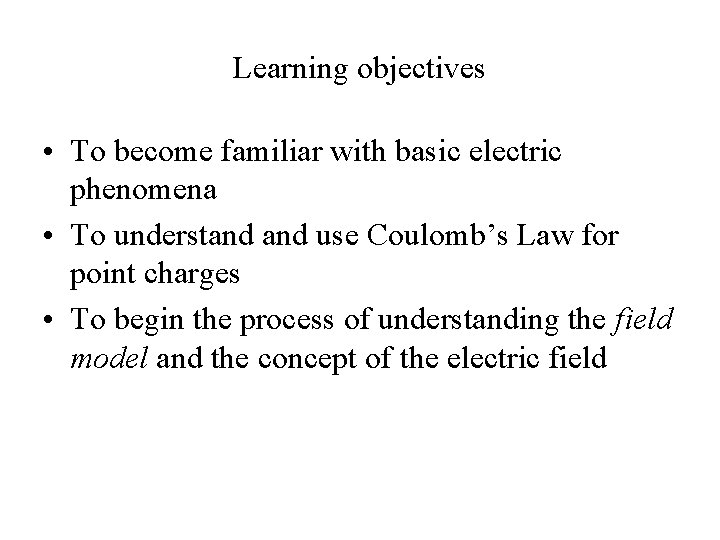Learning objectives • To become familiar with basic electric phenomena • To understand use Coulomb’s Law for point charges • To begin the process of understanding the field model and the concept of the electric field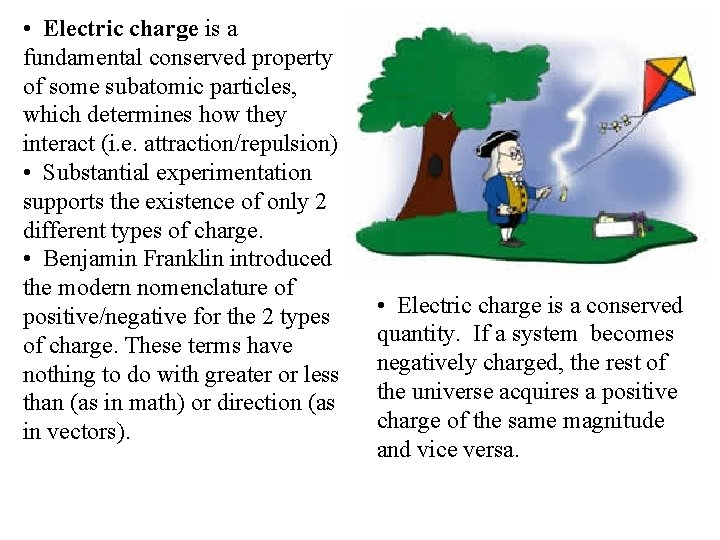• Electric charge is a fundamental conserved property of some subatomic particles, which determines how they interact (i. e. attraction/repulsion) • Substantial experimentation supports the existence of only 2 different types of charge. • Benjamin Franklin introduced the modern nomenclature of positive/negative for the 2 types of charge. These terms have nothing to do with greater or less than (as in math) or direction (as in vectors). • Electric charge is a conserved quantity. If a system becomes negatively charged, the rest of the universe acquires a positive charge of the same magnitude and vice versa.• Familiar charged particles include the proton and the electron. • The SI unit of electric charge is the Coulomb (C). • A Coulomb is a lot of charge! The charge of one proton or electron is: • Objects become charged when they have an excess or a deficit of electrons, which are mobile. • Like charges (pos/pos or neg/neg) repel each other • Unlike charges attract. • Neutral objects are attracted to both kinds of charge. That will be explained later in the chapter.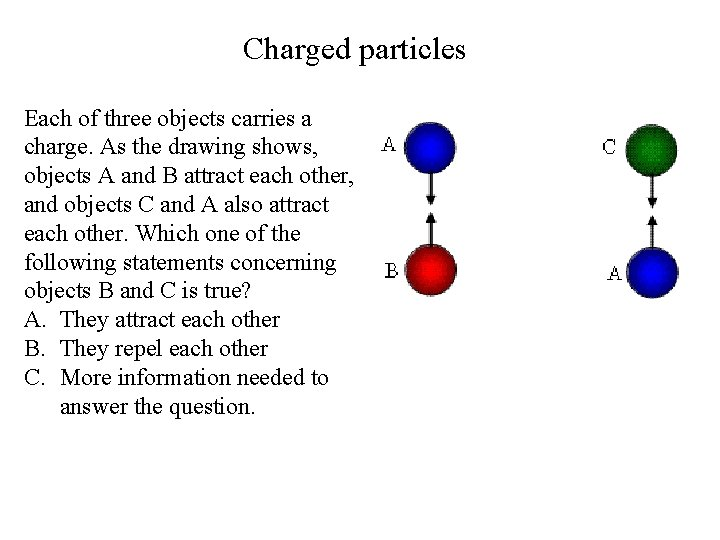Charged particles Each of three objects carries a charge. As the drawing shows, objects A and B attract each other, and objects C and A also attract each other. Which one of the following statements concerning objects B and C is true? A. They attract each other B. They repel each other C. More information needed to answer the question.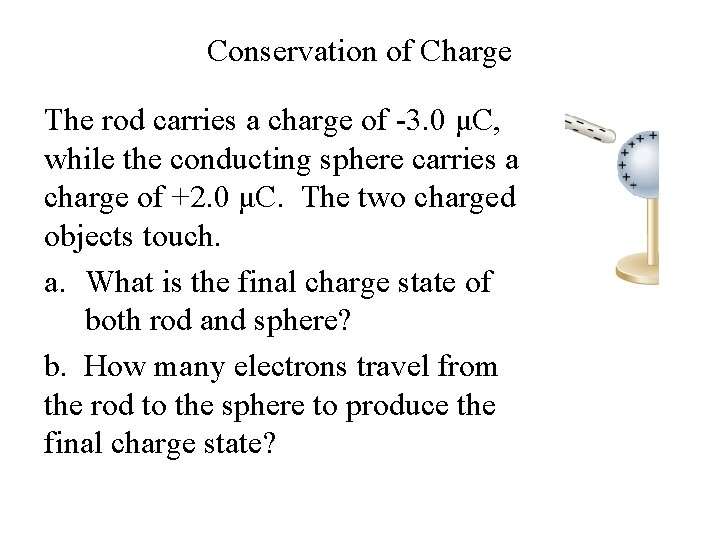Conservation of Charge The rod carries a charge of -3. 0 μC, while the conducting sphere carries a charge of +2. 0 μC. The two charged objects touch. a. What is the final charge state of both rod and sphere? b. How many electrons travel from the rod to the sphere to produce the final charge state?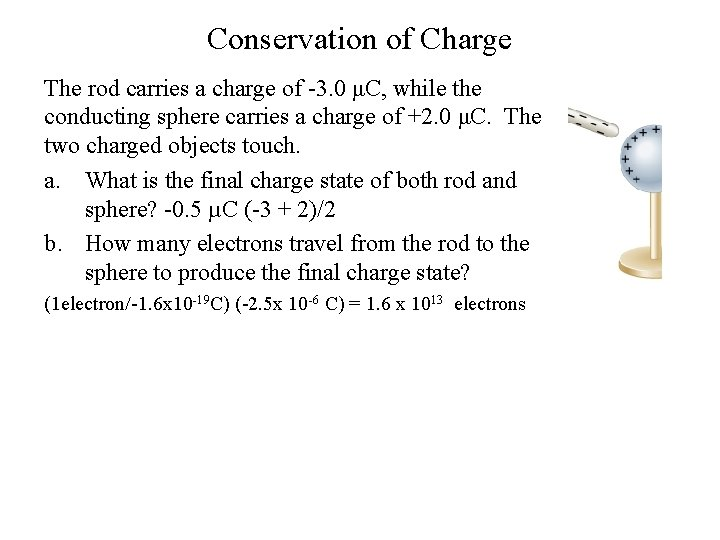Conservation of Charge The rod carries a charge of -3. 0 μC, while the conducting sphere carries a charge of +2. 0 μC. The two charged objects touch. a. What is the final charge state of both rod and sphere? -0. 5 µC (-3 + 2)/2 b. How many electrons travel from the rod to the sphere to produce the final charge state? (1 electron/-1. 6 x 10 -19 C) (-2. 5 x 10 -6 C) = 1. 6 x 1013 electrons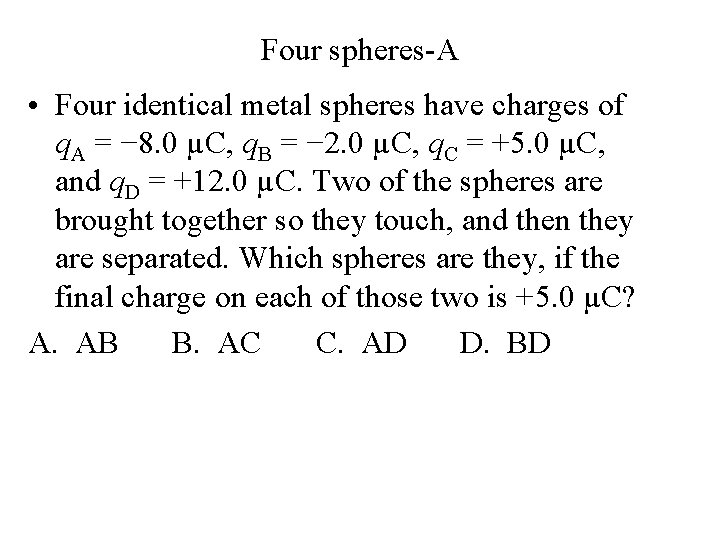Four spheres-A • Four identical metal spheres have charges of q. A = − 8. 0 µC, q. B = − 2. 0 µC, q. C = +5. 0 µC, and q. D = +12. 0 µC. Two of the spheres are brought together so they touch, and then they are separated. Which spheres are they, if the final charge on each of those two is +5. 0 µC? A. AB B. AC C. AD D. BD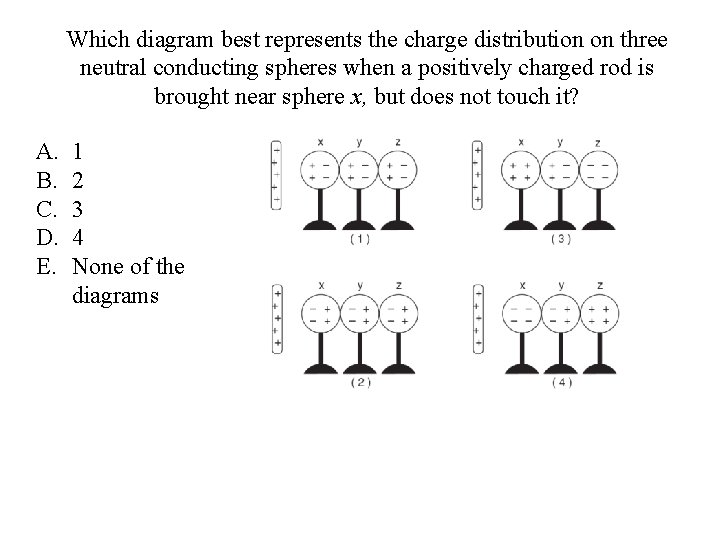Which diagram best represents the charge distribution on three neutral conducting spheres when a positively charged rod is brought near sphere x, but does not touch it? A. B. C. D. E. 1 2 3 4 None of the diagrams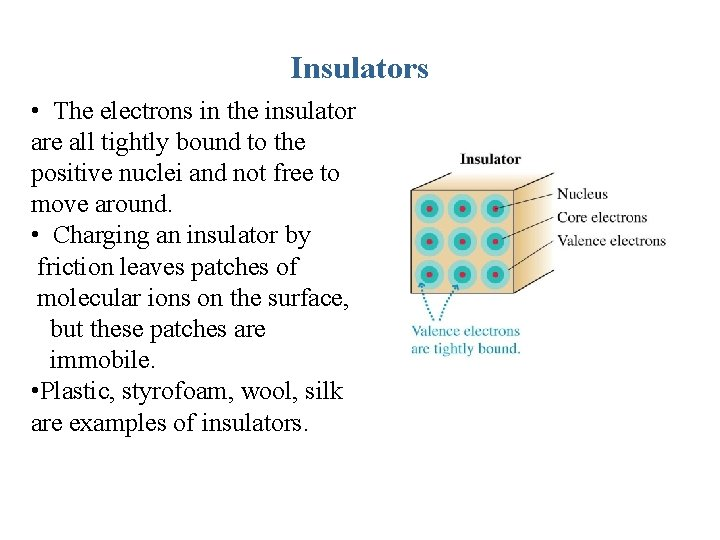Insulators • The electrons in the insulator are all tightly bound to the positive nuclei and not free to move around. • Charging an insulator by friction leaves patches of molecular ions on the surface, but these patches are immobile. • Plastic, styrofoam, wool, silk are examples of insulators.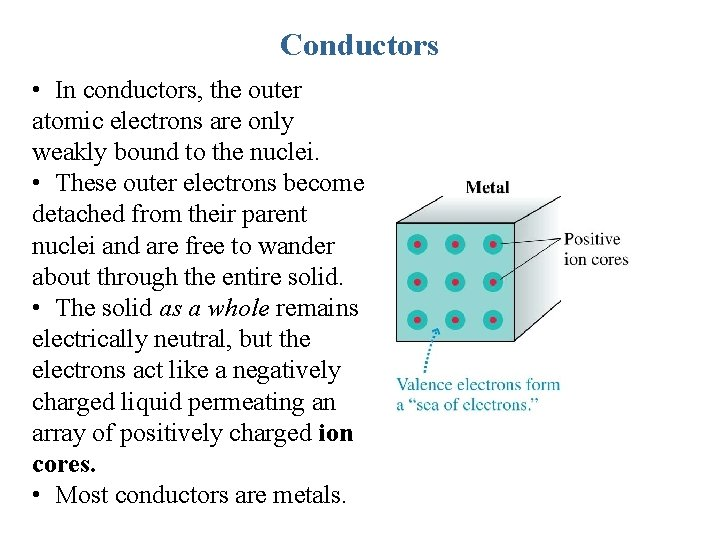Conductors • In conductors, the outer atomic electrons are only weakly bound to the nuclei. • These outer electrons become detached from their parent nuclei and are free to wander about through the entire solid. • The solid as a whole remains electrically neutral, but the electrons act like a negatively charged liquid permeating an array of positively charged ion cores. • Most conductors are metals.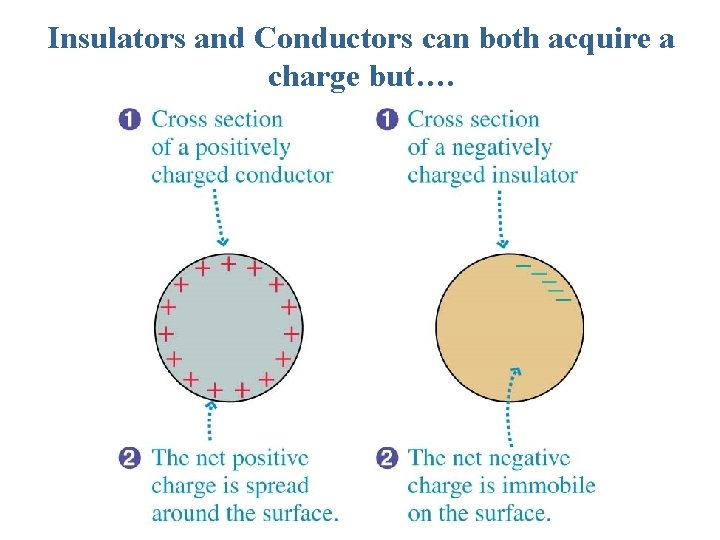Insulators and Conductors can both acquire a charge but….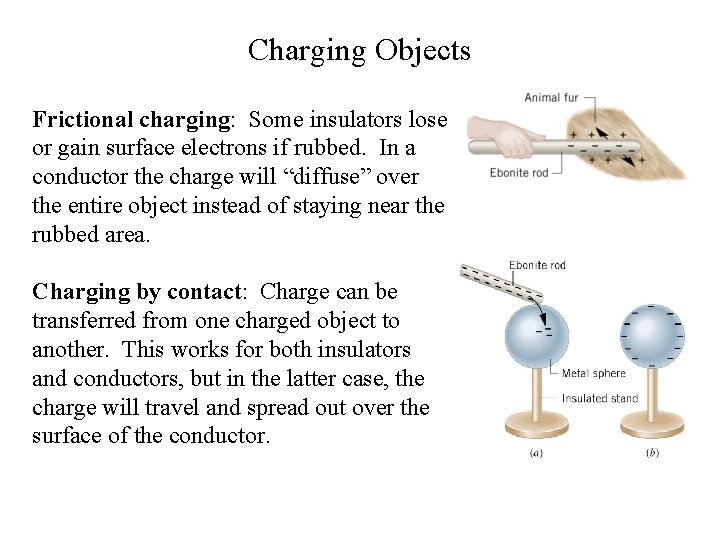Charging Objects Frictional charging: Some insulators lose or gain surface electrons if rubbed. In a conductor the charge will “diffuse” over the entire object instead of staying near the rubbed area. Charging by contact: Charge can be transferred from one charged object to another. This works for both insulators and conductors, but in the latter case, the charge will travel and spread out over the surface of the conductor.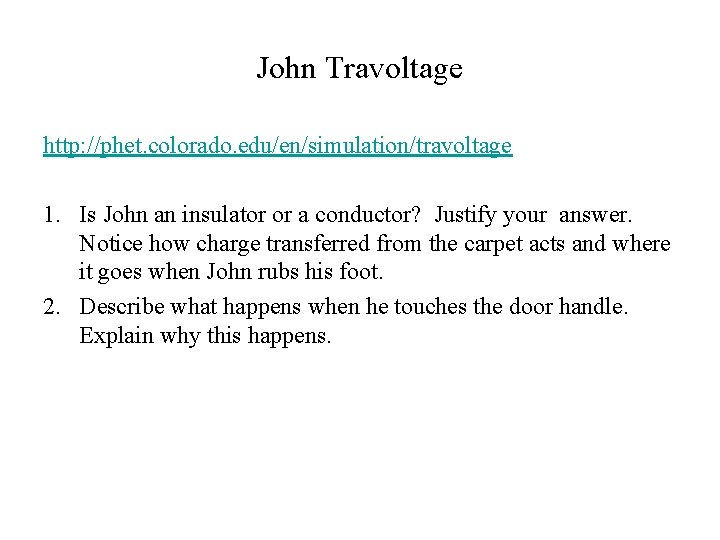John Travoltage http: //phet. colorado. edu/en/simulation/travoltage 1. Is John an insulator or a conductor? Justify your answer. Notice how charge transferred from the carpet acts and where it goes when John rubs his foot. 2. Describe what happens when he touches the door handle. Explain why this happens.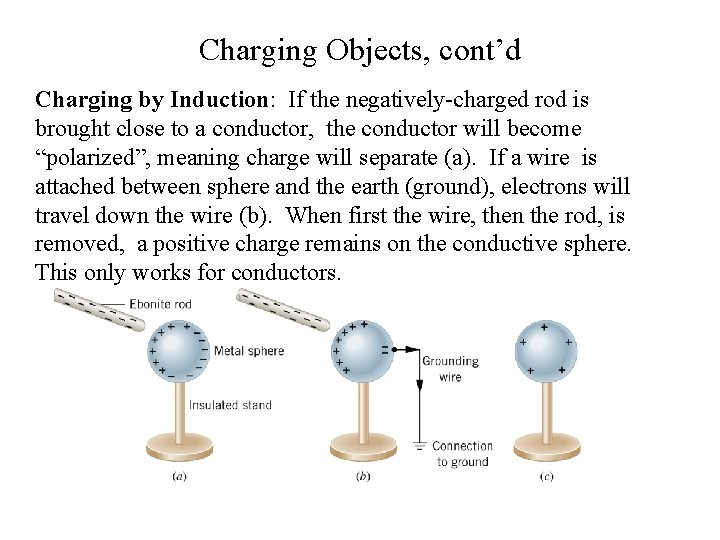Charging Objects, cont’d Charging by Induction: If the negatively-charged rod is brought close to a conductor, the conductor will become “polarized”, meaning charge will separate (a). If a wire is attached between sphere and the earth (ground), electrons will travel down the wire (b). When first the wire, then the rod, is removed, a positive charge remains on the conductive sphere. This only works for conductors.Stop to Think: Is Order Important? What would happen if, in situation (b) below, we took the rod away first, then disconnected the grounding wire? A. The metal sphere would still have a positive charge, because the order of events doesn’t matter B. The metal sphere would remain neutral because with the rod gone, the electrons that went down the wire would travel back up the grounding wire C. The metal sphere would become negative because once the rod is gone, too many electrons would travel up the grounding wire.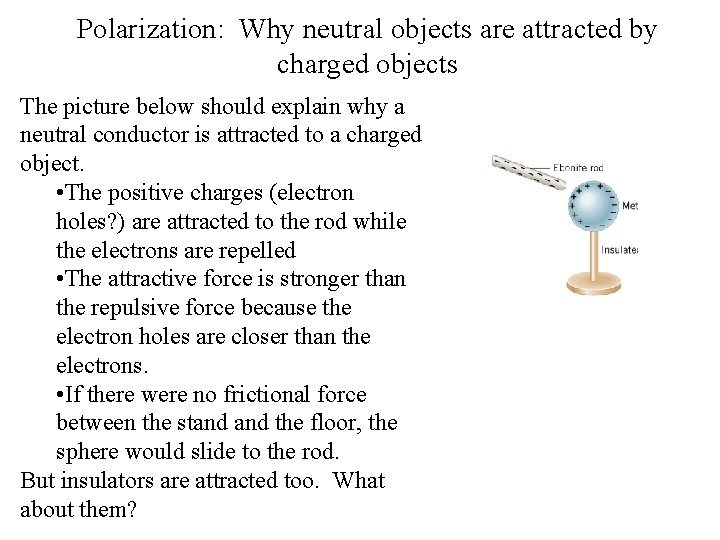Polarization: Why neutral objects are attracted by charged objects The picture below should explain why a neutral conductor is attracted to a charged object. • The positive charges (electron holes? ) are attracted to the rod while the electrons are repelled • The attractive force is stronger than the repulsive force because the electron holes are closer than the electrons. • If there were no frictional force between the stand the floor, the sphere would slide to the rod. But insulators are attracted too. What about them?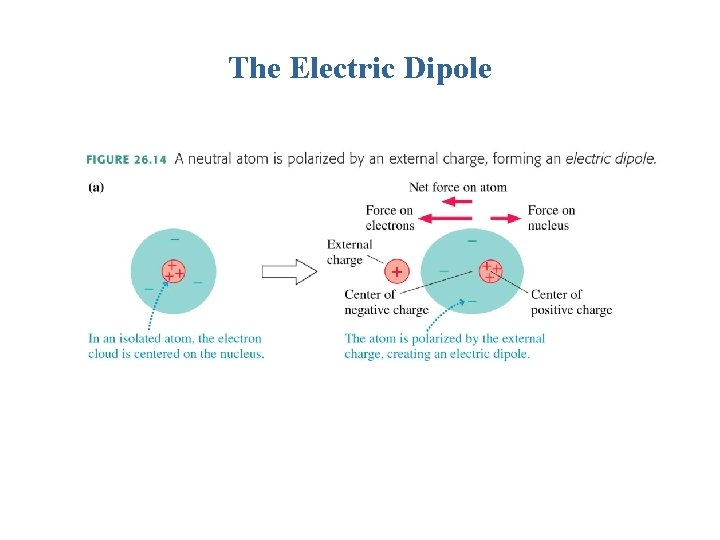The Electric Dipole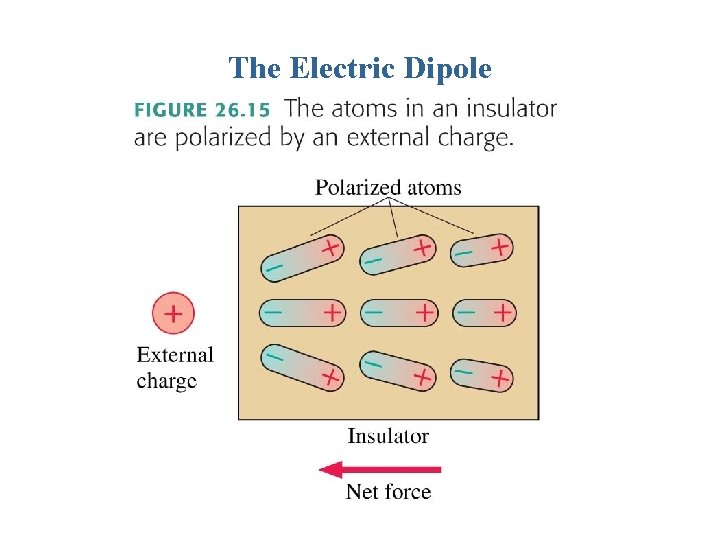The Electric Dipole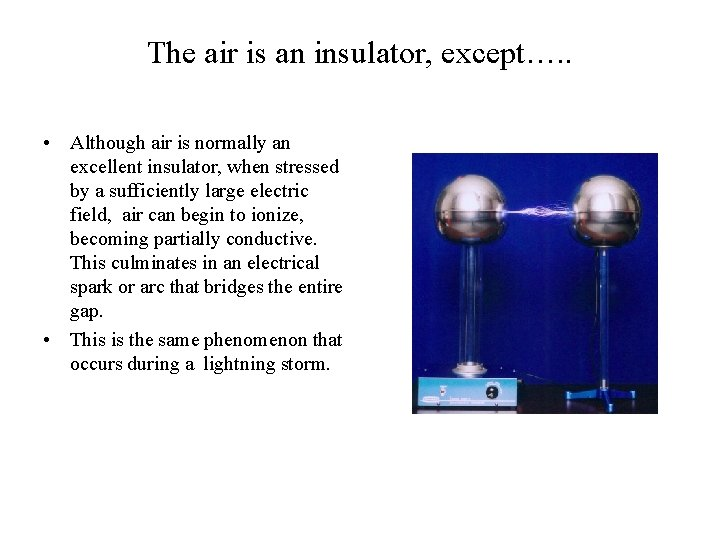The air is an insulator, except…. . • Although air is normally an excellent insulator, when stressed by a sufficiently large electric field, air can begin to ionize, becoming partially conductive. This culminates in an electrical spark or arc that bridges the entire gap. • This is the same phenomenon that occurs during a lightning storm.COULOMB’S LAW In SI units k = 8. 99 × 109 N m 2/C 2. k = 1/4πε 0, where ε 0 is called the permittivity of free space and equals 8. 85 x 10 -12 C 2/(N m 2). Note: Assume the weight force is negligible unless otherwise specified in problems and diagrams.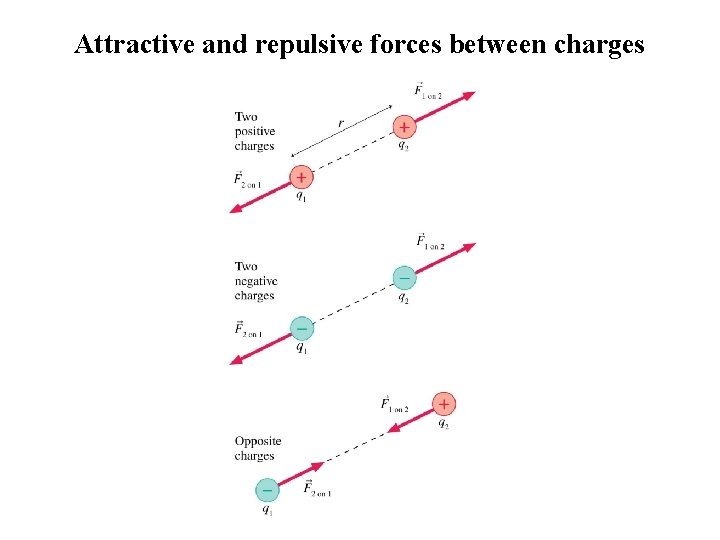Attractive and repulsive forces between chargesCharges A and B exert repulsive forces on each other. q. A = 4 q. B. Which statement is true? A. FA on B > FB on A B. FA on B < FB on A C. FA on B = FB on A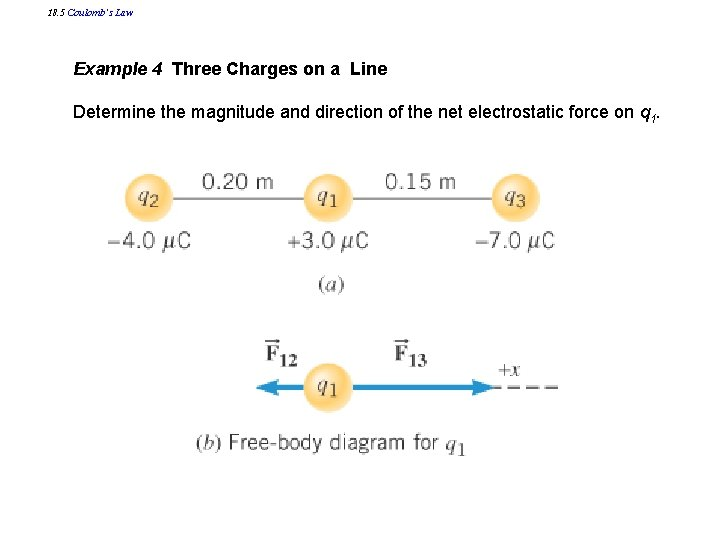18. 5 Coulomb’s Law Example 4 Three Charges on a Line Determine the magnitude and direction of the net electrostatic force on q 1.18. 5 Coulomb’s Law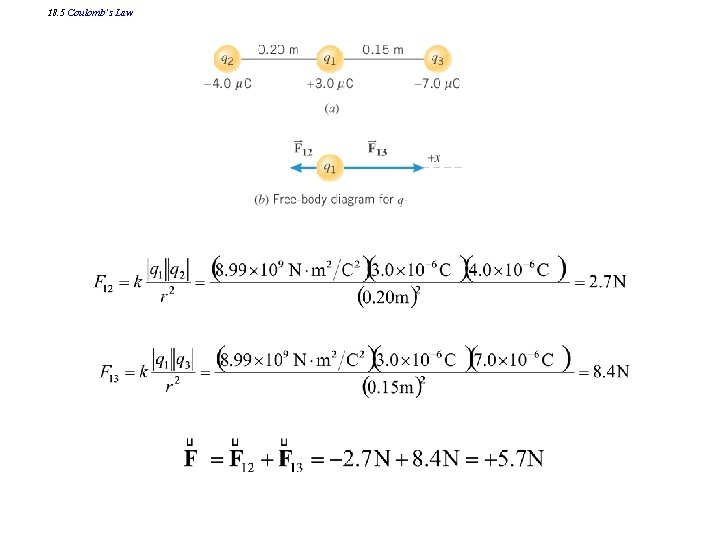18. 5 Coulomb’s Law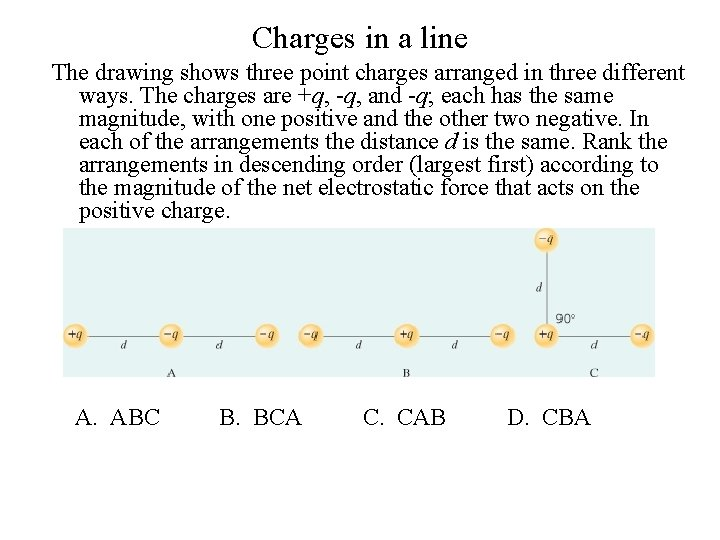Charges in a line The drawing shows three point charges arranged in three different ways. The charges are +q, -q, and -q; each has the same magnitude, with one positive and the other two negative. In each of the arrangements the distance d is the same. Rank the arrangements in descending order (largest first) according to the magnitude of the net electrostatic force that acts on the positive charge. ABC B. BCA C. CAB D. CBA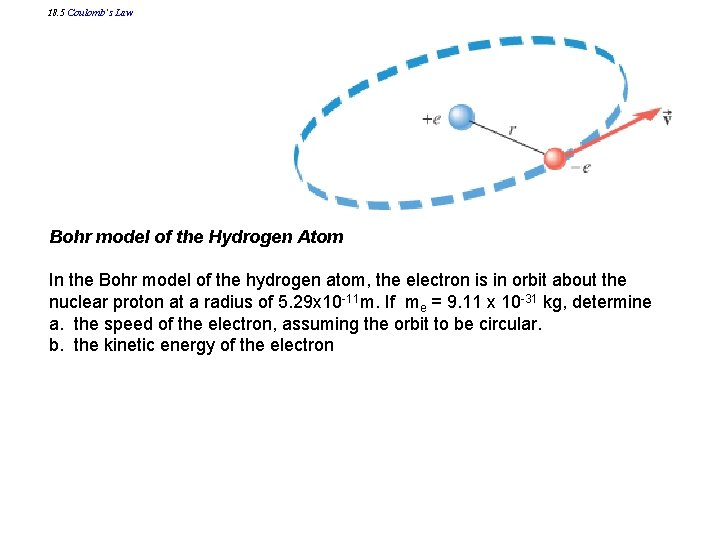18. 5 Coulomb’s Law Bohr model of the Hydrogen Atom In the Bohr model of the hydrogen atom, the electron is in orbit about the nuclear proton at a radius of 5. 29 x 10 -11 m. If me = 9. 11 x 10 -31 kg, determine a. the speed of the electron, assuming the orbit to be circular. b. the kinetic energy of the electron18. 5 Coulomb’s Law 1. Draw a fbd of the electron (ignore weight force, even though mass is used) 2. Use Newton’s 2 nd Law: ΣF = ma. In this case, there is only the electrical force, and a is a centripetal acceleration; a = v 2/r and18. 5 Coulomb’s Law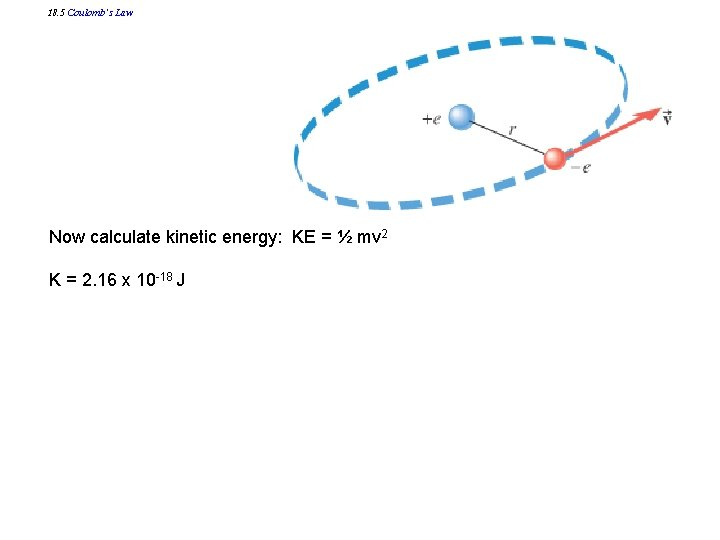18. 5 Coulomb’s Law Now calculate kinetic energy: KE = ½ mv 2 K = 2. 16 x 10 -18 JThe Electric Field Model: Newton vs Faraday • In this illustration, consider A to be one or more source charges, in that it is the source of the “alteration of space”. • Consider B to be the test charge (q 0), a small visitor to the neighborhood.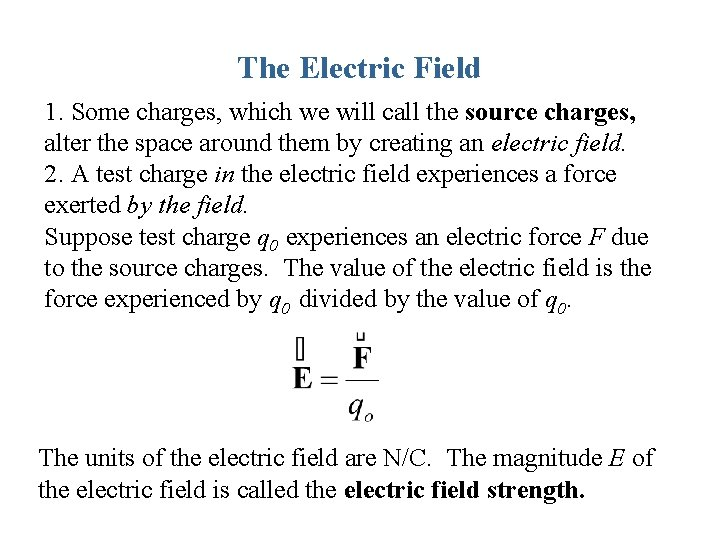The Electric Field 1. Some charges, which we will call the source charges, alter the space around them by creating an electric field. 2. A test charge in the electric field experiences a force exerted by the field. Suppose test charge q 0 experiences an electric force F due to the source charges. The value of the electric field is the force experienced by q 0 divided by the value of q 0. The units of the electric field are N/C. The magnitude E of the electric field is called the electric field strength.The Electric Field The electric field vector points in the same directions as that of the electrostatic force on a positive test charge • The electric field vector points in the opposite direction from the electrostatic force on a negative test charge • The electric field due to one or more source charges exists in all space whethere is a test charge or not http: //phet. colorado. edu/en/simulation/electric-hockey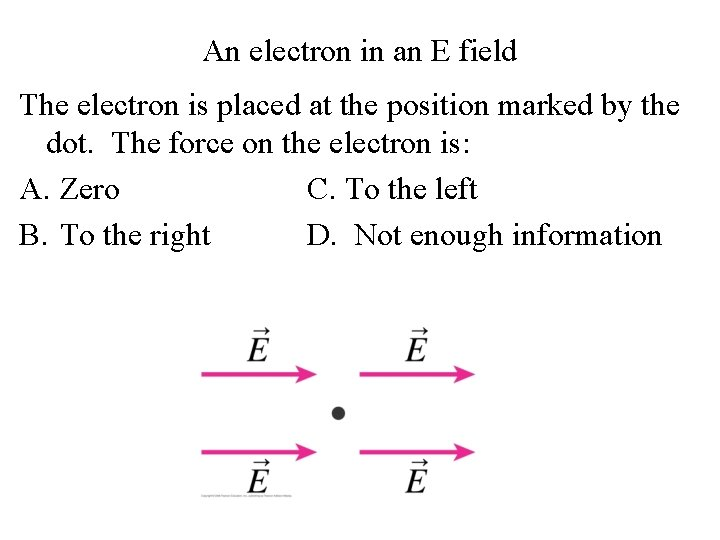An electron in an E field The electron is placed at the position marked by the dot. The force on the electron is: A. Zero C. To the left B. To the right D. Not enough information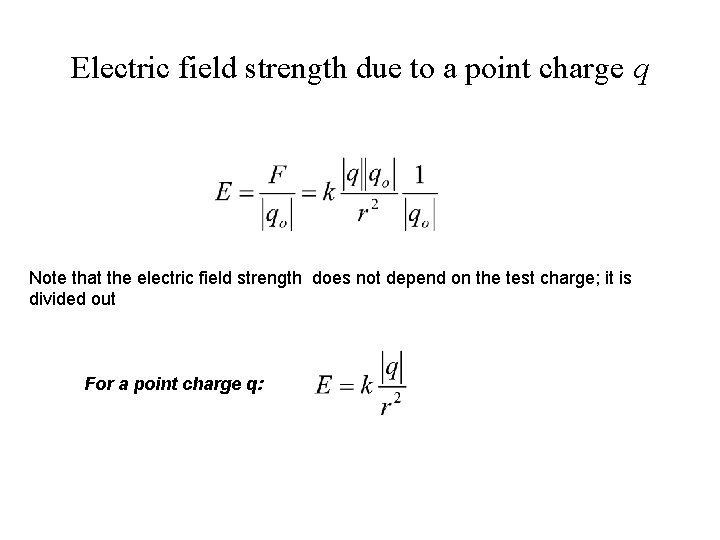Electric field strength due to a point charge q Note that the electric field strength does not depend on the test charge; it is divided out For a point charge q:Example Problem Two charges are placed on the x axis. One of the charges (q 1 = +9. 1 µC) is at x 1 = +2. 8 cm and the other (q 2 = -22 µC) is at x 2 = +8. 7 cm. Find the net electric field (magnitude and direction) at x = 0 cm. (Use the sign of your answer to indicate the direction along the xaxis. ). In SI units k = 8. 99 × 109 N m 2/C 2. 1 cm = 10 -2 mExample Problem Two charges are placed on the x axis. One of the charges (q 1 = +9. 1 µC) is at x 1 = +2. 8 cm and the other (q 2 = -22 µC) is at x 2 = +8. 7 cm. Find the net electric field (magnitude and direction) at x = 0 cm. (Use the sign of your answer to indicate the direction along the xaxis. ). In SI units k = 8. 99 × 109 N m 2/C 2. 1 cm = 10 -2 m Ans: -7. 82 x 107 N/CElectric field strength Rank electric field strengths at points a, b, c, d from greatest to least:The E field vector The electric field vector at 1 can be represented by the arrow shown above. How long are the arrows at 2 and 4? Explain your reasoning.c. If the distance between the unseen point charge and the 900 N/C point is r 1, and the distance between the unseen point charge and the 400 N/C is r 2, by what factor is r 2 > r 1 ? Explain your reasoning.THE PARALLEL PLATE CAPACITOR σ = q/A = charge density • The capacitor is a chargestorage device used in many electrical appliances and circuits. • The simplest type is the parallel plate capacitor, two metal plates of area A. • Each plate has an equal amount of charge, with one being positive and the other negative. • The electric field inside the capacitor is constant and does not depend on the distance from the plates.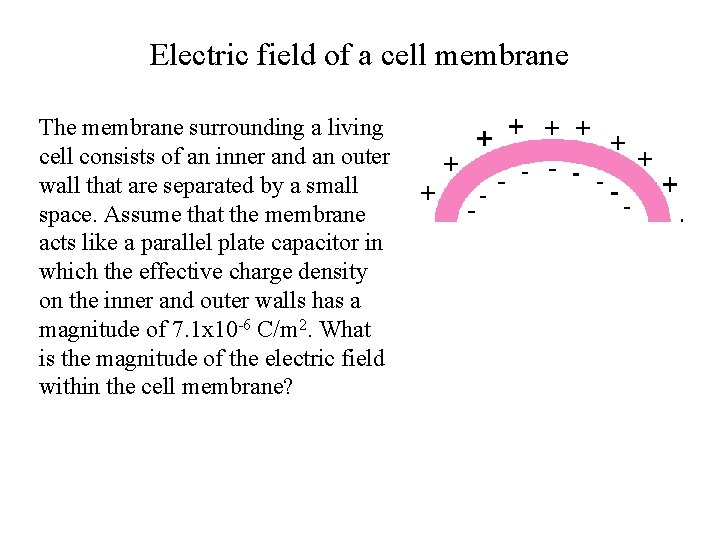Electric field of a cell membrane The membrane surrounding a living cell consists of an inner and an outer wall that are separated by a small space. Assume that the membrane acts like a parallel plate capacitor in which the effective charge density on the inner and outer walls has a magnitude of 7. 1 x 10 -6 C/m 2. What is the magnitude of the electric field within the cell membrane?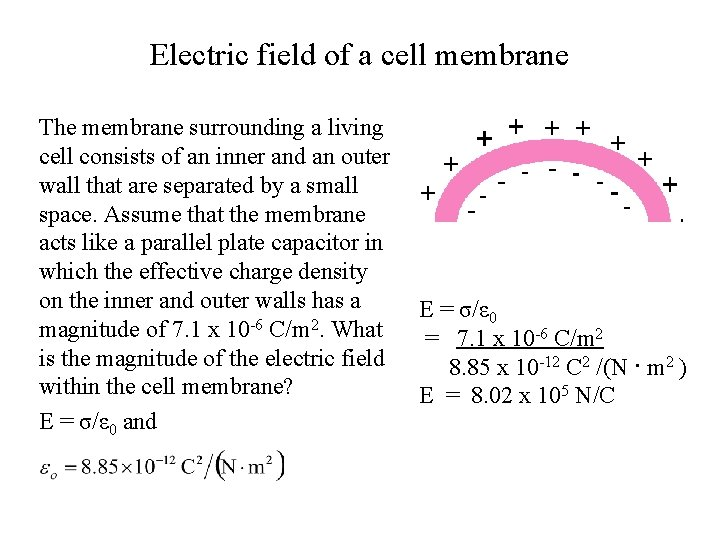Electric field of a cell membrane The membrane surrounding a living cell consists of an inner and an outer wall that are separated by a small space. Assume that the membrane acts like a parallel plate capacitor in which the effective charge density on the inner and outer walls has a magnitude of 7. 1 x 10 -6 C/m 2. What is the magnitude of the electric field within the cell membrane? E = σ/ε 0 and E = σ/ε 0 = 7. 1 x 10 -6 C/m 2 8. 85 x 10 -12 C 2 /(N · m 2 ) E = 8. 02 x 105 N/C18. 7 Electric Field Lines Electric field lines or lines of force provide a map of the electric field in the space surrounding electric charges. Figure a shows electric field vectors. Figure b shows the electric field lines due to a positive point charge source.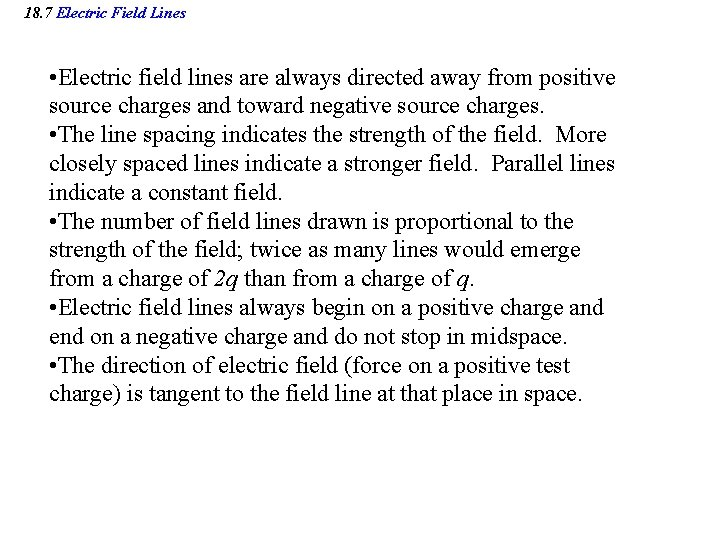18. 7 Electric Field Lines • Electric field lines are always directed away from positive source charges and toward negative source charges. • The line spacing indicates the strength of the field. More closely spaced lines indicate a stronger field. Parallel lines indicate a constant field. • The number of field lines drawn is proportional to the strength of the field; twice as many lines would emerge from a charge of 2 q than from a charge of q. • Electric field lines always begin on a positive charge and end on a negative charge and do not stop in midspace. • The direction of electric field (force on a positive test charge) is tangent to the field line at that place in space.Electric field lines due to a point charge Positive Negative Electric field lines are always directed away from positive source charges and toward negative source charges. Lines are more closely spaced close to the charge, where the field is stronger.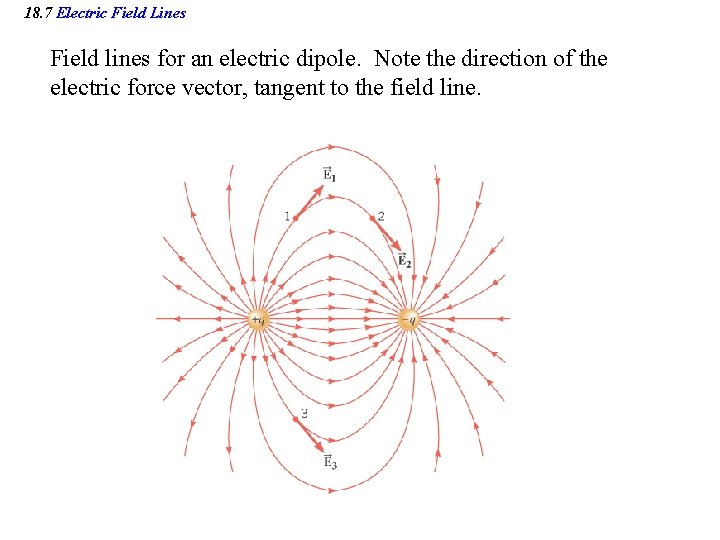18. 7 Electric Field Lines Field lines for an electric dipole. Note the direction of the electric force vector, tangent to the field line.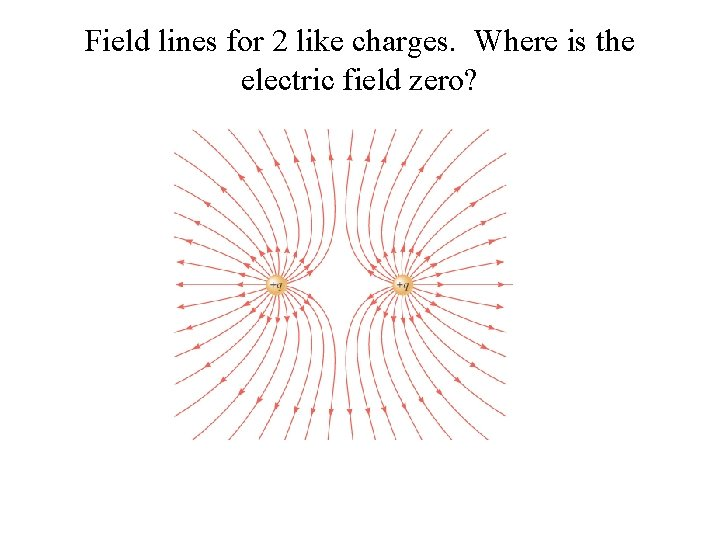Field lines for 2 like charges. Where is the electric field zero?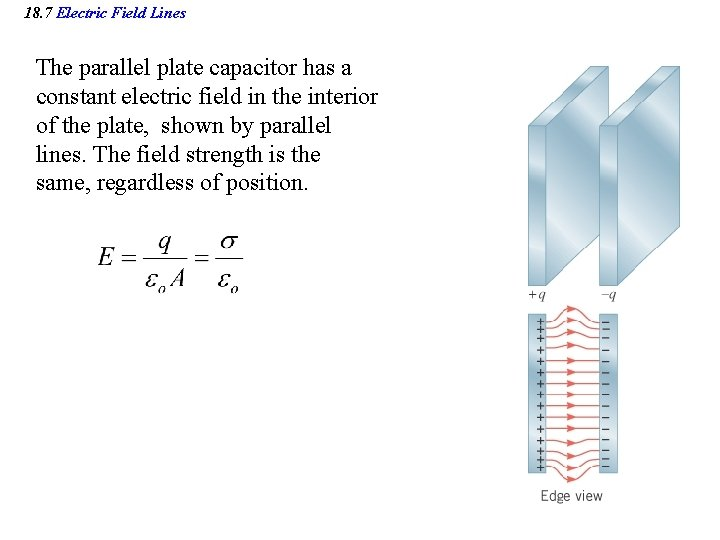18. 7 Electric Field Lines The parallel plate capacitor has a constant electric field in the interior of the plate, shown by parallel lines. The field strength is the same, regardless of position.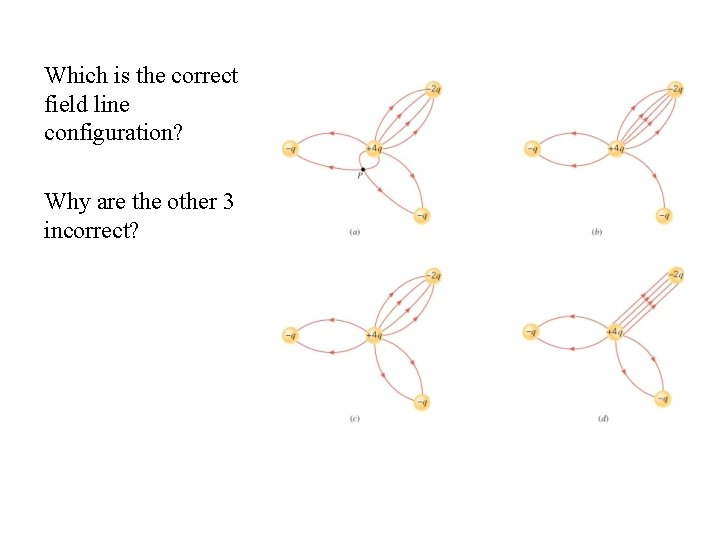Which is the correct field line configuration? Why are the other 3 incorrect?Focus on Concepts The drawing shows some electric field lines. For the points indicated, rank the magnitudes of the electric field in descending order (largest first). A. BCA C. CAB E. ABC B. BAC D. ACB